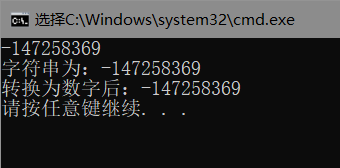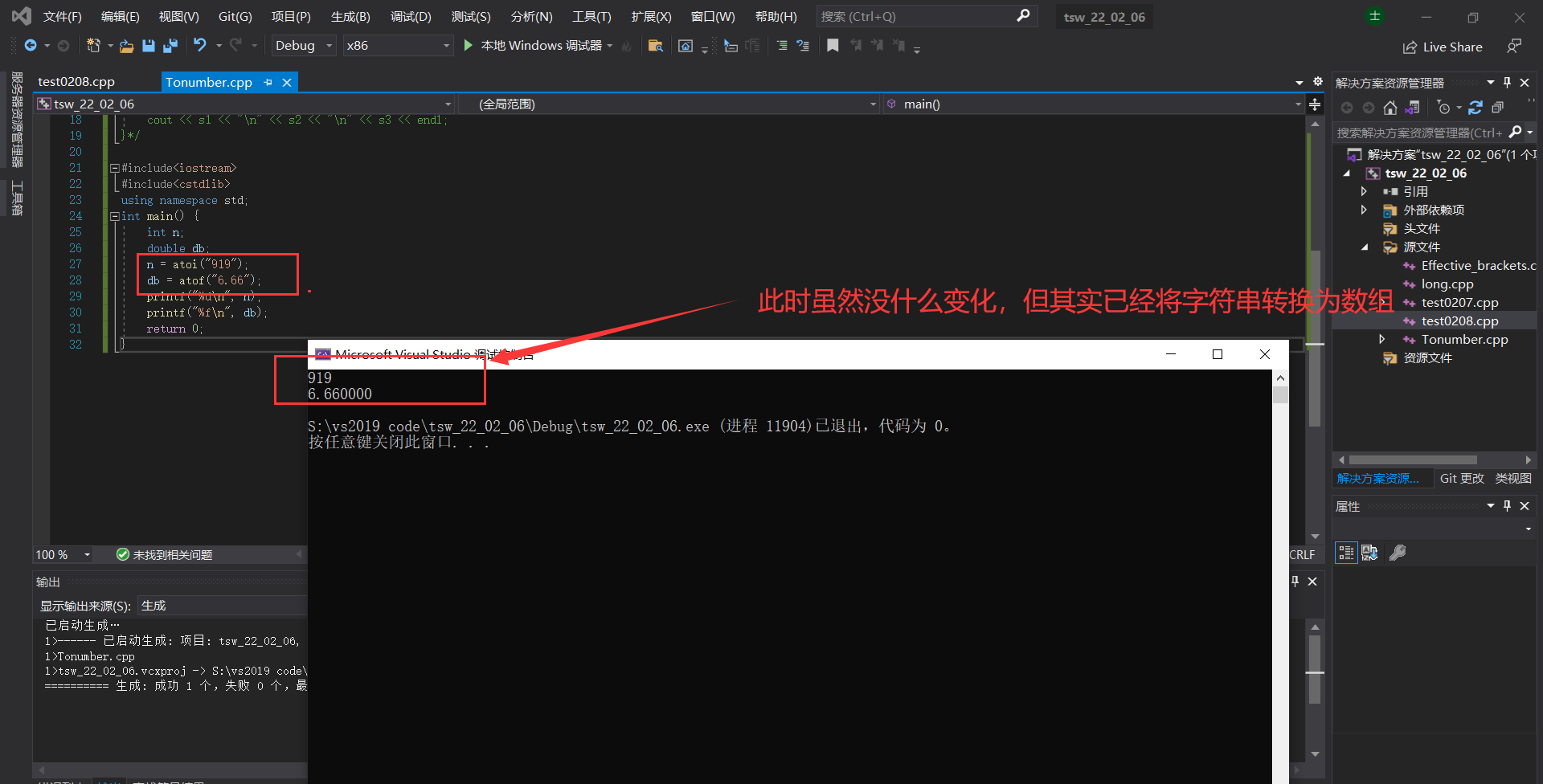• C语言中将字符串转换为数字
万次阅读 多人点赞
2020-11-06 11:51:44

函数名 作 用

## atof() 将字符串转换为双精度浮点型值 atoi() 将字符串转换为整型值 atol() 将字符串转换为长整型值 strtod() 将字符串转换为双精度浮点型值，并报告不能被转换的所有剩余数字 strtol() 将字符串转换为长整值，并报告不能被转换的所有剩余数字 strtoul() 将字符串转换为无符号长整型值，并报告不能被转换的所有剩余数字

（1）下面的实例演示了 atof() 函数的用法。

#include <stdio.h>
#include <stdlib.h>
#include <string.h>

int main()
{
float val;
char str;

strcpy(str, "98993489");
val = atof(str);
printf("字符串值 = %s, 浮点值 = %f\n", str, val);

strcpy(str, "runoob");
val = atof(str);
printf("字符串值 = %s, 浮点值 = %f\n", str, val);

return(0);
}


编译并运行上面的程序，产生以下结果：

字符串值 = 98993489, 浮点值 = 98993488.000000
字符串值 = runoob, 浮点值 = 0.000000


（2）以下是用atoi()函数将字符串转换为整数的例子：

# include <stdio. h>
# include <stdlib. h>
void main (void) ;
void main (void)
{
int num;
char * str = "100";
num = atoi(str);
printf("字符串值 = %s, 整型值 = %d\n",str, num);
}


让我们编译并运行上面的程序，这将产生以下结果：

字符串值 =100, 整型值 = 100


atoi()函数只有一个参数，即要转换为数字的字符串str。atoi()函数的返回值就是转换所得的整型值num。c语言
更多相关内容
• 主要介绍了C语言实现将字符串转换为数字的方法,涉及系统函数atoi()函数的使用技巧,需要的朋友可以参考下
• 今天小编就大家分享一篇Python不使用int()函数把字符串转换为数字的方法，具有很好的参考价值，希望对大家有所帮助。一起跟随小编过来看看吧
• number_format（）函数用于将字符串转换为数字。它会在成功时返回格式化的数字，否则会在失败时给出E_WARNING。 <?php \$num = "1000.314"; // 使用number_format()函数将字符串转换为数字 echo number_format...
• C++字符串转化为数字的库函数 1、atoi 功 能:把一字符串转换为整数 用 法:int atoi(const char *nptr); 详细解释:atoi是英文array to integer 的缩写。atoi()会扫描参数nptr字符串，如果第一个字符不是数字也不是...
• 今天小编就大家分享一篇vue 实现强制类型转换 数字类型转为字符串，具有很好的参考价值，希望对大家有所帮助。一起跟随小编过来看看吧
• ## 字符串转换为数字

千次阅读 2019-09-28 21:32:39
问题描述：输入一个表示整数的字符串，把该字符串转换成整数并输出。例如，输入字符串“12345”，输出整数“12345”。 解决方法：依次扫描字符串，遇数字就把在之前得到的数字乘以10再加上当前字符串表示的数字。但...

声明：题目来源于《王道》

问题描述：输入一个表示整数的字符串，把该字符串转换成整数并输出。例如，输入字符串“12345”，输出整数“12345”。

解决方法：依次扫描字符串，遇数字就把在之前得到的数字乘以10再加上当前字符串表示的数字。但需考虑特殊情况，即整数可能不仅仅只含有数字，还可能会有“+”、“-”符号来表示数的正负。如果是“+”，则不需要做处理，如果是“-”，则表明此数为负数。接着还要考虑非法输入，若输入的字符串中还有不是数字的字符，则每当遇到这样的字符时就停止转换，还有，若转换结果，溢出，则返回0。

#include <iostream>
#include <algorithm>
using namespace std;
int StrToInt(char* str)
{
long long num = 0;
if (str != NULL)
{
char* digit = str;
bool minus = false;		//负数标记位
if (*digit == '+')
{
digit++;
}
else if (*digit == '-')
{
digit++;
minus = true;
}
while (*digit != '\0')
{
if (*digit >= '0' && *digit <= '9')		//如果是数字，转
{
num = num * 10 + (*digit - '0');
if (num > numeric_limits<int>::max())		//溢出，返回0，停止转换
{
num = 0;
break;
}
digit++;
}
else		//如果有非数字字符，停止转换，返回0
{
num = 0;
break;
}
}
if (*digit == '\0')
{
if (minus)
{
num = 0 - num;
}
}
}
return num;
}

int main()
{
char s;
cin >> s;
cout << "字符串为：" << s << endl;
cout << "转换为数字后：" << StrToInt(s) << endl;
return 0;
}展开全文• 例如：这个判断基偶性就是为了防止越界所以将数组转换为数组传入，再将最后一位字母转换数字判断其基偶性 #include<iostream> using namespace std; int main() { int n; cin >> n; string s; ...

C++中将字符串转换为数字
法一：

int t = s[len - 1]-'0';//减去一个  '0'  是
将最后一位字母转换成数字


例如：这个判断基偶性就是为了防止越界所以将数组转换为数组传入，再将最后一位字母转换位数字判断其基偶性

#include<iostream>
using namespace std;
int main() {
int n;
cin >> n;
string s;
while (n--) {
cin >> s;
int len = s.length();
int t = s[len - 1]-'0';//减去一个  '0'  是将最后一位字母转换成数字
if (t % 2 == 0) {
cout << "even" << endl;

}
else
cout << "odd" << endl;

}
}


法二：

#include<iostream>
#include<cstdlib>
using namespace std;
int main() {
int n;
double db;
n = atoi("919");
db = atof("6.66");
printf("%d\n", n);
printf("%f\n", db);
return 0;
}展开全文c++ 开发语言 后端
• ecvt()：将双精度浮点型值转换为字符串转换结果中不包含十进制小数点。 fcvt()：指定位数为转换精度，其余同ecvt()。 这里只进行演示了itoa 和 gcvt。 # include int main () { int num_int = 435; double num_...

# 1.stringstream方法

记得头文件添加sstream

#include <iostream>
#include <string.h>
#include<sstream>
using namespace std;
int main()
{
string a="1234";
stringstream geek(a);
int b;
geek>>b;
cout<<b+5<<endl;
return 0;
}


# 2.-'0’法

number = number*10+a[i]-‘0’;

#include <iostream>
using namespace std;
int main()
{
string a="1234";
int l = a.length();
int b[l];
for(int i=0; i<l; i++)
{
b[i]=a[i]-'0';
}

for(int i=0; i<l; i++)
{
cout<<b[i];
}
}


# 3.标准库函数

itoa()：将整型值转换为字符串。
ltoa()：将长整型值转换为字符串。
ultoa()：将无符号长整型值转换为字符串。
gcvt()：将浮点型数转换为字符串，取四舍五入。
ecvt()：将双精度浮点型值转换为字符串，转换结果中不包含十进制小数点。
fcvt()：指定位数为转换精度，其余同ecvt()。

这里只进行演示了itoa 和 gcvt。

# include <stdio.h>

int main ()
{
int num_int = 435;
double num_double = 435.10001f;
char str_int;
char str_double;

itoa(num_int, str_int, 10);  //10代表十进制的结果进行转换
gcvt(num_double,5,str_double);  //5为精确位数

printf("str_int: %s\n", str_int);
printf("str_double: %s\n", str_double);

return 0;
}


# 4.sscanf 与 sprintf

超级好用噻 假设现在有个字符数组str;
语法结构:
sscanf(str，“%d”，&n)；//把字符数组str中的内容以"%d"格式输入到n中。
sprintf(str,"%d",n);//把n中的数以"%d"的形式传送到str字符数组中。
下面是例子：
sscanf //别忘记取地址符号

# include <stdio.h>
# include <stdlib.h>

int main ()
{
char str="123";
int n;
sscanf(str,"%d",&n);
printf("%d",n);
return 0;
}


ssprintf

# include <stdio.h>
# include <stdlib.h>

int main ()
{
char str;
int n=123;
sprintf(str,"%d",n);
printf("%s",str);
return 0;
}

展开全文c++
• 在编程中我们经常需要进行各种数据类型之间的转换操作，下面将您介绍如何将字符串转换为数字。首先我们需要了解各种数据类型的表示，String在java中为字符串类型，int在java中为整数类型，大小在-2147483648到...
• 先介绍三个库函数：atoi、atol、atof，可分别把ASCII编码的字符串转化为int、long、float类型的数字。 头文件：stdlib.h 对于整型（int型） 对于 0～9之间的数字基础方法 原理：ASCII码中，字符‘0’~‘9’对应的...c语言
• 字符串转化为数字的python实现将字符串转化为数字的python实现，例如将字符串“1234567.8”转化 1234567.8这也是学习python中的一个简单的练习题，代码如下：# coding=UTF-8 将字符串转化为数字from functools ...
• 字符串转换为数字 public static void main(String[] args) { String str1="1234"; String str2="123456789"; String str3="1234.12"; String str4="1234.5678"; String str5="210102199906180520908007"; //转换...java
• 说明：在实际的应用过程中，有的时候可能会遇到字符串的10，需要将字符串的10转换为数字的10在此记录下，通过int函数转换的过程。操作过程：1.将字符串转换为整型的10>>> str1 = "10" #将一个字符串的10赋...
• 本文主要讲了C语言中将数字转换为字符串的方法，希望对你的学习有所帮助。
• 主要介绍了详解C++中十六进制字符串数字（数值）的相关资料,这里提供两种实现方法，需要的朋友可以参考下
• 方法主要有三种 转换函数、强制类型转换、利用js变量弱类型转换。 1. 转换函数： js提供了parseInt()和parseFloat()两个转换函数。前者把值转换成整数，后者把值转换成浮点数。只有对String类型调用这些方法，这两个...
• If a String was to be built like: 连接String时，还有一种更巧妙的方法来转换数字。 如果要像这样构建字符串： String aboutDog = "My dog is " + numberTwenty + " the conversion of the int numberTwenty is ...java python 编程语言 c++
• ## C语言将字符串转换为数字

万次阅读 多人点赞 2020-04-02 11:17:00
最近做了一些需要使用字符串形势获取数字的程序，这里就牵扯到了字符串类型和数字类型的转换问题，以下是C语言中，字符串类型转换为数字类型的方法。 1.问题描述 数字既能以字符串形式储存，也能以数值形式储存，把...c语言
• 方法一：使用列表推导式 >>> a = ['1', '2', '3', '4', '5'] >>> b = list(int(x) for x in a) >>> b [1, 2, 3, 4, 5] 方法二：内置函数map() >>>...方法三：python
• 实现了将数字字符串转化为单个数字的源码，已测试可用
• 题目描述：实现类似c中atoi()函数的功能，将一个字符串转化为数字。如字符串"123"转化123.需要考虑的问题：1). 字符串第一位有没有符号，即+,-; 这里假设没有（如果有符号，还需要考虑若只有一个符号的...
• 【函数说明】atoi() 函数会扫描参数 str 字符串，跳过前面的空白字符（例如空格，tab缩进等，可以通过 isspace() 函数来检测），直到遇上数字或正负符号才开始做转换，而再遇到非数字字符串结束时(’\0’)才结束...
• 字符串转化为数字的python实现将字符串转化为数字的python实现，例如将字符串“1234567.8”转化 1234567.8这也是学习python中的一个简单的练习题，代码如下：# coding=UTF-8 将字符串转化为数字from functools ...
• 通过转换说明吧数字数字形式转换为字符串形式；scanf()函数把输入字符串转换为数值形式；应用场景：编写程序需要使用数值命令形参，但是命令形参被读取为字符串。要使用数值必须先把字符串转换为数字。atoi()函数...
• 函数的功能字符串转化为整数 提示：仔细思考所有可能的输入情况。这个问题没有给出输入的限制，你需要自己考虑所有可能的情况。 输入 "123" 返回值 123 分析 1.对字符串进行判空处理，如果字符串空，则...
• C语言提供了几个标准库函数，可以将字符串转换为任意类型(整型、长整型、浮点型等)的数字。以下是用atoi()函数将字符串转换为整数的一个例子：# include <stdio. h># include <stdlib. h>void main ...
• C++代码： #include<iostream> #include<cmath> using namespace std; int atoi(const char *str) { if (str == NULL) { return 0; } int n = strlen(str), i = 0; bool flag = true;...c++
• 使用 map() 字符串 => 数字： const arr = ['1', '2', '3'] arr.map(Number) // [1, 2, 3] 数字 => 字符串 const arr = [1, 2, 3] arr.map(String) // ['1', '2', '3']javascript 前端...